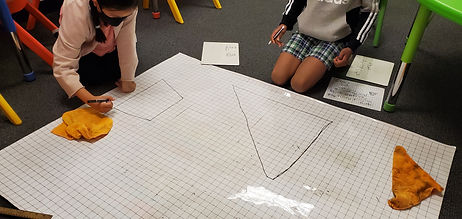Mr. Jake

Target 1​

Lesson Type:

New

Measurment

:

Techniques and Formulas

Solve problems involving area.

1:

Find the area of triangles, trapezoids, and parallelograms.

2:

Use previous area understandings (of rectangular figures) to gain insight into the area of new geometric shapes.

3:

Determine the algebraic equations for calculating the area of a triangle (½ base * height), a trapezoid (½ height * (base1 + base2)), and a parallelogram (base * height).

4:

Calculate the area of a shaded region of a figure.

8th

Vocabulary:

Trapezoid, Area, Varible, Formula

Activities:

Calculated the area of trapezoidal by breaking them down into multiple shapes and using the formula

Used the formula to find the missing base or height measurements using the given area.Home Exploration

Guiding Questions:Absent Students:

Target 2

:

Vocabulary:

Activities:Home Exploration

Guiding Questions:Target 3

:

Vocabulary:

Activities:Home Exploration